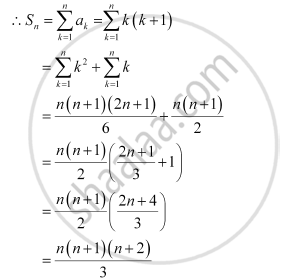CBSE (Arts) Class 11CBSE
Share
Notifications

View all notifications
Books Shortlist
Your shortlist is empty

# Find the Sum to N Terms of the Series 1 × 2 + 2 × 3 + 3 × 4 + 4 × 5 + … - CBSE (Arts) Class 11 - Mathematics

Login
Create free account

Forgot password?
ConceptSum to N Terms of Special Series

#### Question

Find the sum to n terms of the series 1 × 2 + 2 × 3 + 3 × 4 + 4 × 5 + …

#### Solution

The given series is 1 × 2 + 2 × 3 + 3 × 4 + 4 × 5 + …

nth term, an = n ( n + 1)Is there an error in this question or solution?

#### APPEARS IN

NCERT Solution for Mathematics Textbook for Class 11 (2018 to Current)
Chapter 9: Sequences and Series
Q: 1 | Page no. 196
Solution Find the Sum to N Terms of the Series 1 × 2 + 2 × 3 + 3 × 4 + 4 × 5 + … Concept: Sum to N Terms of Special Series.
S